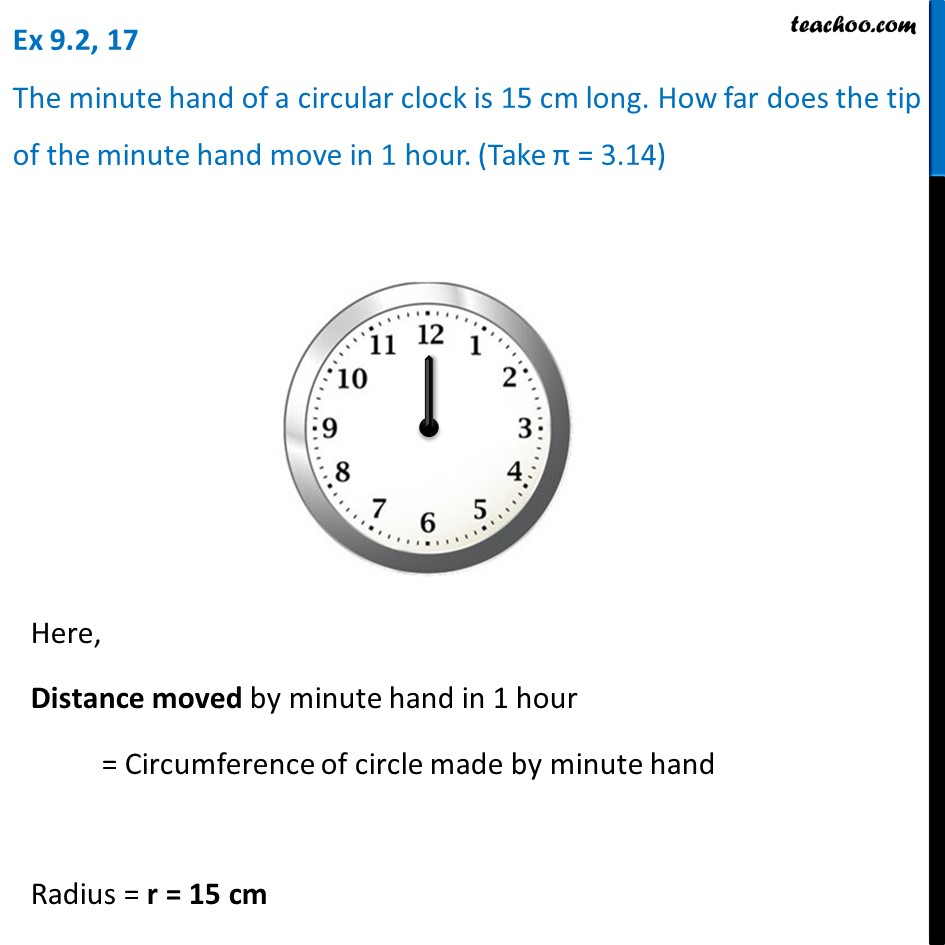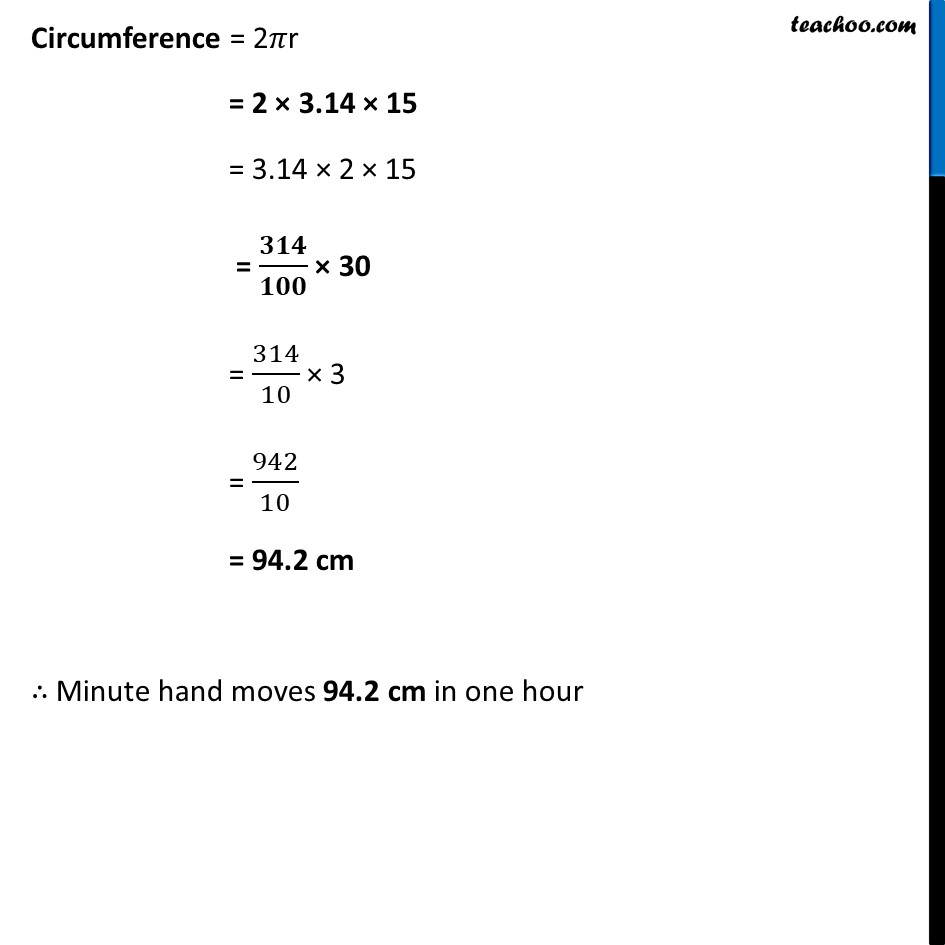Ex 9.2

Chapter 9 Class 7 Perimeter and Area
Serial order wiseLearn in your speed, with individual attention - Teachoo Maths 1-on-1 Class

### Transcript

Ex 9.2, 17 The minute hand of a circular clock is 15 cm long. How far does the tip of the minute hand move in 1 hour. (Take π = 3.14) Here, Distance moved by minute hand in 1 hour = Circumference of circle made by minute hand Radius = r = 15 cm Circumference = 2𝜋r = 2 × 3.14 × 15 = 3.14 × 2 × 15 = 𝟑𝟏𝟒/𝟏𝟎𝟎 × 30 = 314/10 × 3 = 942/10 = 94.2 cm ∴ Minute hand moves 94.2 cm in one hour